# Mathematical Extensions

Currently the operators and basic predicates of the Event-B mathematical language supported by Rodin are fixed. We propose to extend Rodin to define basic predicates, new operators or new algebraic types.

## Requirements

### User Requirements

• Binary operators (prefix form, infix form or suffix form).
• Operators on boolean expressions.
• Unary operators, such as absolute values.
Note that the pipe, which is already used for set comprehension, cannot be used to enter absolute values (in fact, as in the new design the pipe used in set comprehension is only a syntaxic sugar, the same symbol may be used for absolute value. To be confirmed with the prototyped parser. It may however be not allowed in a first time until backtracking is implemented, due to use of lookahead making assumptions on how the pipe symbol is used. -Mathieu ).
• Basic predicates (e.g., the symmetry of relations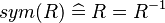$sym(R) \defi R=R^{-1}$).
Having a way to enter such predicates may be considered as syntactic sugar, because it is already possible to use sets (e.g.,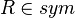$R \in sym$, where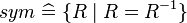$sym \defi \{R \mid R=R ^{-1}\}$) or functions (e.g.,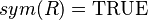$sym(R) = \True$, where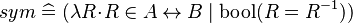$sym \defi (\lambda R \qdot R \in A \rel B \mid \bool(R = R^{-1}))$).
• Quantified expressions (e.g.,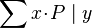$\sum x \qdot P \mid y$,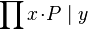$\prod x \qdot P \mid y$,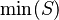$~\min(S)$,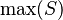$~\max(S)$).
• Types.
• Enumerated types.
• Scalar types.

### User Input

The end-user shall provide the following information:

• keyboard input
• Lexicon and Syntax.
More precisely, it includes the symbols, the form (prefix, infix, postfix), the grammar, associativity (left-associative or right associative), commutativity, priority, the mode (flattened or not), ...
• Pretty-print.
Alternatively, the rendering may be determined from the notation parameters passed to the parser.
• Typing rules.
• Well-definedness.

• Scalability.

## Towards a generic AST

The following AST parts are to become generic, or at least parameterised:

• Lexer
• Parser
• Nodes ( Formula class hierarchy ): parameters needed for:
• Type Solve (type rule needed to synthesize the type)
• Type Check (type rule needed to verify constraints on children types)
• WD (WD predicate)
• PrettyPrint (tag image + notation (prefix, infix, postfix) + needs parentheses (deduced from compatibility graph))
• Visit Formula (getting children + visitor callback mechanism)
• Rewrite Formula (associative formulæ have a specific flattening treatment)
• Types (Type class hierarchy): parameters needed for:
• Building the type expression (type rule needed)
• PrettyPrint (set operator image)
• getting Base / Source / Target type (type rule needed)
• Verification of preconditions (see for example AssociativeExpression.checkPreconditions)

### Vocabulary

An extension is to be understood as a single additional operator definition.

### Tags

Every extension is associated with an integer tag, just like existing operators. Thus, questions arise about how to allocate new tags and how to deal with existing tags.
The solution proposed here consists in keeping existing tags 'as is'. They are already defined and hard coded in the Formula class, so this choice is made with backward compatibility in mind.

Now concerning extension tags, we will first introduce a few hypotheses:

• Tags_Hyp1: tags are never persisted across sessions on a given platform
• Tags_Hyp2: tags are never referenced for sharing purposes across various platforms

In other words, cross-platform/session formula references are always made through their String representation. These assumptions, which were already made and verified for Rodin before extensions, lead us to restrict further considerations to the scope of a single session on a single platform.

The following definitions hold at a given instant$t$ and for the whole platform.
Let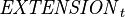$\mathit{EXTENSION}_t$ be the set of extensions supported by the platform at instant$t$;
let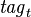$\mathit{tag}_t$ denote the affectation of tags to an extension at instant$t$ (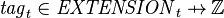$\mathit{tag}_t \in \mathit{EXTENSION}_t \pfun \intg$);
let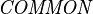$\mathit{COMMON}$ be the set of existing tags defined by the Formula class (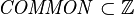$\mathit{COMMON} \sub \intg$).
The following requirements emerge:

• Tags_Req1: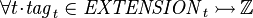$\forall t \qdot \mathit{tag}_t \in \mathit{EXTENSION}_t \tinj \intg$
• Tags_Req2: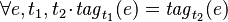$\forall e, t_1,t_2 \qdot \mathit{tag}_{t_1}(e)=\mathit{tag}_{t_2}(e)$ where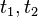$t_1, t_2$ are two instants during a given session
• Tags_Req3: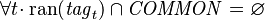$\forall t \qdot \ran(\mathit{tag}_t) \cap \mathit{COMMON} = \empty$

The above-mentioned scope-restricting hypothesis can be reformulated into: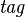$\mathit{tag}$ needs not be stable across sessions nor across platforms.

### Formula Factory

The requirements about tags give rise to a need for centralising the$\mathit{tag}$ relation in order to enforce tag uniqueness. The Formula Factory appears to be a convenient and logical candidate for playing this role. Each time an extension is used to make a formula, the factory is called and it can check whether its associated tag exists, create it if needed, then return the new extended formula while maintaining tag consistency.

The factory can also provide API for requests about tags and extensions: getting the tag from an extension and conversely.

We also need additional methods to create extended formulæ. A first problem to address is: which type should these methods return ? We could define as many extended types as the common AST API does, namely ExtendedUnaryPredicate, ExtendedAssociativeExpression, and so on, but this would lead to a large number of new types to deal with (in visitors, filters, …), together with a constraint about which types extensions would be forced to fit into. It is thus preferable to have as few extended types as possible, but with as much parameterisation as can be. Considering that the two basic types Expression and Predicate have to be extensible, we come to add two extended types ExtendedExpression and ExtendedPredicate.

ExtendedExpression makeExtendedExpression( ? )
ExtendedPredicate makeExtendedPredicate( ? )


Second problem to address: which arguments should these methods take ? Other factory methods take the tag, a collection of children where applicable, and a source location. In order to discuss what can be passed as argument to make extended formulæ, we have to recall that the make… factory methods have various kinds of clients, namely:

• parser
• POG
• provers

(other factory clients use the parsing or identifier utility methods: SC modules, indexers, …)

Thus, the arguments should be convenient for clients, depending on which information they have at hand. The source location does not obviously seem to require any adaptation and can be taken as argument the same way. Concerning the tag, it depends on whether clients have a tag or an extension at hand. Both are intended to be easily retrieved from the factory. As a preliminary choice, we can go for the tag and adjust this decision when we know more about client convenience.

As for children, the problem is more about their types. We want to be able to handle as many children as needed, and of all possible types. Looking to existing formula children configurations, we can find:

• expressions with predicate children: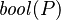$\mathit{bool}(P)$
• expressions with expression children: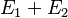$E_1 + E_2$
• predicates with predicate children: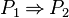$P_1 \limp P_2$
• predicates with expression children: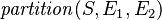$\mathit{partition}(S, E_1, E_2)$
• mixed operators: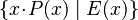$\{x \qdot P(x) \mid E(x)\}$, but it is worth noting that the possibility of introducing bound variables in extended formulæ is not established yet.

Thus, for the sake of generality, children of both types should be supported for both extended predicates and extended expressions.

ExtendedExpression makeExtendedExpression(int tag, Expression[] expressions, Predicate[] predicates, SourceLocation location)
ExtendedPredicate makeExtendedPredicate(int tag, Expression[] expressions, Predicate[] predicates, SourceLocation location)


## Defining Extensions

An extension is meant to contain every information and behaviour required by:

• Keyboard
• (Extensible Fonts ?)
• Lexer
• Parser
• AST
• Static Checker
• Proof Obligation Generator
• Provers

### Keyboard requirements

Kbd_req1: an extension must provide an association combo/translation for every uncommon symbol involved in the notation.


### Lexer requirements

Lex_req1: an extension must provide an association lexeme/token for every uncommon symbol involved in the notation.


### Parser requirements

According to the Parser page, the following informations are required by the parser in order to be able to parse a formula containing a given extension.

• symbol compatibility
• group compatibility
• symbol precedence
• group precedence
• notation:
• prefix, infix, …
• configuration (sub-parsers, symbols and their relative position, child types)

### AST requirements

#### Operator Properties API

Operator properties are reified through the following interfaces and enumerated:

#### Extension API

Extensions are required to implement the following APIs in order to be supported in the AST. It is the expression of the missing information for a ExtendedExpression (resp. ExtendedPredicate) to behave as a Expression (resp. Predicate). It can also be viewed as the parametrization of the AST.

#### Mediator API

The mediators provided as arguments to these APIs have the following specification:

#### Example: Binary PLUS

public class BinaryPlus implements IExpressionExtension {

public Type getType(ITypeMediator mediator, ExtendedExpression expression) {
final Type resultType = mediator.makeIntegerType();
for (Expression child : expression.getChildExpressions()) {
final Type childType = child.getType();
if (!childType.equals(resultType)) {
return null;
}
}
return resultType;
}

public Type typeCheck(ITypeCheckMediator mediator,
ExtendedExpression expression) {
final Type resultType = mediator.makeIntegerType();
for (Expression child : expression.getChildExpressions()) {
mediator.sameType(child.getType(), resultType);
}
return resultType;
}

public String getSyntaxSymbol() {
return "+";
}

public Predicate getWDPredicate(IWDMediator mediator,
IExtendedFormula formula) {
return mediator.makeTrueWD();
}

}

// no priorities to set
}

public String getGroupId() {
return "arithmetic";
}

public String getId() {
return "binary plus";
}

public ExtensionKind getKind() {
return ExtensionKind.BINARY_INFIX_EXPRESSION;
}

}


### Static Checker requirements

The static checker is involved in:

• static checking an extension definition (pre deployment)
TODO
• static checking a model that uses extensions (post deployment);
to that purpose, (deployed) extensions need to be placed in the build dependency graph before models that use them
it must check that referenced (deployed) extensions are present in the project
it needs to get the appropriate FormulaFactory for parsing (with referenced extensions enabled)
it must be aware of extensions being added/deleted/replaced to reprocess dependent models

### Proof Obligation Generator requirements

Likewise, the POG may run on

• an extension definition (pre deployment)
TODO
• a model that uses extensions (post deployment)
nothing to do for WD POs (relies on the fact that extension WD is transmitted to the AST)

TODO

TODO

## User Input Summarization

Identified required data entail the following user input:

• id:
a String id
• group id:
an identifier of a existing group a new group id
• kind (determines both the type and the notation):
a choice between
• PARENTHESIZED_PREFIX_PREDICATE: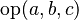$\operatorname{op}(a,b,c)$
• PARENTHESIZED_PREFIX_EXPRESSION: idem
• BINARY_INFIX_EXPRESSION: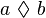$a ~ \lozenge ~ b$
• ASSOCIATIVE_INFIX_EXPRESSION: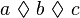$a ~ \lozenge ~ b ~ \lozenge ~ c$

As a first step we may restrict the choice to the above list. For all these kinds, all children are expressions.

• syntax symbol:
a String equal to the operator to be parsed in formulæ (for instance "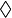$\lozenge$" or "myOperator" or "\u03d5")
• keyboard:
a list of associations 'symbol <-> ASCII shortcut' for uncommon symbols
• parsing:
compatibility:
• a list of operator ids of the same group, that are compatible with the defined operator
in particular, if the id of the defined operator is included, it means it is compatible with itself
priority:
• a list of other operator ids of the same group with higher priority than this operator
• a list of other operator ids of the same group with lower priority than this operator
additionally, if a new group is defined, it shall be given
• the id of another group with lower priority
• the id of another group with higher priority
As these informations have a global scope, we may think about more convenient ways to input/review them, avoiding a manual scanning of all individual extensions.
• type rule:
• the type of every child
as a first step we may restrict children to all bear the same type, for instance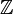$\Z$ or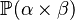$\pow(\alpha \cprod \beta)$.
• (for expressions) the resulting type, which may depend on the type of the children
for instance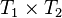$T_1 \cprod T_2$ where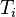$T_i$ stands for the type of the i-th child.
• well-definedness:
as a first step we will only provide a pre-defined WD condition: 'children WD conjunction', so as long as it remains so, nothing needs to be input by the user concerning WD.

## Impact on other tools

Impacted plug-ins (use a factory to build formulæ):

• org.eventb.core
In particular, the static checker and proof obligation generator are impacted.
• org.eventb.core.seqprover
• org.eventb.pp
• org.eventb.pptrans
• org.eventb.ui

## Identified Problems

The static checker shall enforce verifications to detect the following situations:

• Two mathematical extensions are not compatible (the extensions define symbols with the same name but with a different semantics).
• A mathematical extension is added to a model and there is a conflict between a symbol and an identifier.
• An identifier which conflicts with a symbol of a visible mathematical extension is added to a model.

Beyond that, the following situations are problematic:

• A formula has been written with a given parser configuration and is read with another parser configuration.
As a consequence, it appears as necessary to remember the parser configuration.
The static checker will then have a way to invalid the sources of conflicts (e.g., priority of operators, etc).
The static checker will then have a way to invalid the formula upon detecting a conflict (name clash, associativity change, semantic change...) mathieu

• A proof may free a quantified expression which is in conflict with a mathematical extension.
SOLUTION #1: Renaming the conflicting identifiers in proofs?
• A theorem library changes: all proofs made using this library are potentially invalidated
it is just as when a reasoner version changes, proof status should be updated to show broken proofs

## Open Questions

### New types

Which option should we prefer for new types?

• OPTION #1: Transparent mode.
In transparent mode, it is always referred to the base type. As a consequence, the type conversion is implicitly supported (weak typing).
For example, it is possible to define the DISTANCE and SPEED types, which are both derived from the$\intg$ base type, and to multiply a value of the former type with a value of the latter type.
• OPTION #2: Opaque mode.
In opaque mode, it is never referred to the base type. As a consequence, values of one type cannot be converted to another type (strong typing).
Thus, the above multiplication is not allowed.
This approach has at least two advantages:
• Stronger type checking.
• Better prover performances.
• need of extractors to convert back to base types.
• need of extra circuitry to allow things like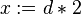$x:=d*2$ where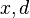$x, d$ are of type DISTANCE
• OPTION #3: Mixed mode.
In mixed mode, the transparent mode is applied to scalar types and the opaque mode is applied to other types.

### Scope of the mathematical extensions

• OPTION #1: Project scope.
The mathematical extensions are implicitly visible to all components of the project that has imported them.
• OPTION #2: Component scope.
The mathematical extensions are only visible to the components that have explicitly imported them. However, note that this visibility is propagated through the hierarchy of contexts and machines (EXTENDS, SEES and REFINES clauses).
An issue has been identified. Suppose that ext1 extension is visible to component C1 and ext2 is visible to component C2, and there is no compatibility issue between ext1 and ext2. It is not excluded that an identifier declared in C1 conflict with a symbol in ext2. As a consequence, a global verification is required when adding a new mathematical extension.
• OPTION #3: Workspace scope.
The mathematical extensions are visible to the whole workspace.

## Examples

### Boolean Operators

Operators "AND" and "OR" are infix associative commutative operators that take two arguments of type BOOL and has a value of type BOOL.

Operator "NOT" is a prefix parenthesized operator that takes one argument of type BOOL and has a value of type BOOL.

They can be defined in a theory as follows:

Similarly, for "OR" and "NOT":

TODO: example of usage in a model

## Bibliography

This proposal consists in considering three kinds of extension:
1. Extensions of set-theoretic expressions or predicates: example extensions of this kind consist in adding the transitive closure of relations or various ordered relations.
2. Extensions of the library of theorems for predicates and operators.
3. Extensions of the Set Theory itself through the definition of algebraic types such as lists or ordered trees using new set constructors.